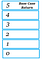# Understanding How Recursion works

`const countUp = num =>{    console.log(`counting...\${num}`);    countUp(num);}countUp(0);`

# Recursion and a Base Case

By passing in an at least one argument which changes with each recursive call we can change the code in the function body itself each time the function calls itself. The key here is the the argument passed in with each recursive call must change in some way with each recursive call. Here’s a simple adjustment to our initial function:

`const countUp = num =>{    console.log(`counting...\${num}`);    countUp(num + 1); // recursive call}countUp(0);`
`const countUp = num =>{    if ( num === 5) return; // base case        console.log(`counting...\${num}`);        countUp(num + 1 ); // recursive call}countUp(0);`

# Order of execution

So far this example isn’t very impressive and only really serves as a replacement of a simple ‘for’ loop, but watch what happens when we move our console.log. Instead of placing our console.log before our recursive call let’s place it after:

`const countUp = num =>{    if ( num === 5) return; // base case        countUp(num + 1 ); // recursive call        console.log(`counting...\${num}`);}countUp(0);`The Execution Stack
`const countUp = num =>{    if ( num === 5){ // base case        console.log(`=== Base case reached (see note B)===`);        return;        }         console.log(`pre-recursive(see note A) - function \${num}`);        countUp(num + 1 ); // recursive call        console.log(`post-recursive(see note C) - function \${num}`);}countUp(1);`
`pre-recursive(see note A) - function 1pre-recursive(see note A) - function 2pre-recursive(see note A) - function 3pre-recursive(see note A) - function 4=== Base case reached (see note B)===post-recursive(see note C) - function 4post-recursive(see note C) - function 3post-recursive(see note C) - function 2post-recursive(see note C) - function 1`
1. The Base Case
2. The Order of recursive execution

Software Developer & Armchair Philosopher

## More from Bobby Valenzuela

Software Developer & Armchair Philosopher